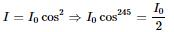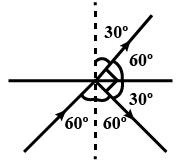Courses

# Test: Polirisation of Light

## 10 Questions MCQ Test Physics For JEE | Test: Polirisation of Light

Description
This mock test of Test: Polirisation of Light for JEE helps you for every JEE entrance exam. This contains 10 Multiple Choice Questions for JEE Test: Polirisation of Light (mcq) to study with solutions a complete question bank. The solved questions answers in this Test: Polirisation of Light quiz give you a good mix of easy questions and tough questions. JEE students definitely take this Test: Polirisation of Light exercise for a better result in the exam. You can find other Test: Polirisation of Light extra questions, long questions & short questions for JEE on EduRev as well by searching above.
QUESTION: 1

### An unpolarised beam of intensity Io is incident on a polarizer and analyser placed in contact. The angle between the transmission axes of the polarizer and the analyser is θ. What is the intensity of light emerging out of the analyser?​

Solution:

Suppose the angle between the transmission axes of the analyser and the polarizer is θ. The completely plane polarized light form the polarizer is incident on the analyser. If E0 is the amplitude of the electric vector transmitted by the polarizer, then intensity I0 of the light incident on the analyser is
I ∞ E02

The electric field vector E0 can be resolved into two rectangular components i.e E0 cosθ and E0 sinθ. The analyzer will transmit only the component ( i.e E0 cosθ ) which is parallel to its transmission axis. However, the component E0sinθ will be absorbed by the analyser. Therefore, the intensity I of light transmitted by the analyzer is,

I ∞ ( E0 x cosθ )2

I / I0 = ( E0 x cosθ )2 / E02 = cos2θ

I = I0 x cos2θ
when light passes from polarizer it's intensity becomes half and when passed through analyser it becomes,
I = I0 x cos2θ/2

QUESTION: 2

### The Brewster’s angle for a transparent medium is 600.The angle of incidence is​

Solution:

Brewster's angle is an angle of incidence at which light with a particular polarization is perfectly transmitted through a transparent dielectric surface, with no reflection. When unpolarized light is incident at this angle, the light that is reflected from the surface is therefore perfectly polarized.
So the angle of incidence would be 60°

QUESTION: 3

### The device or arrangement to detect and check plane polarized light is called

Solution:

An analyser or analyzer is a person or device that analyses given data. It examines in detail the structure of the given data and tries to find patterns and relationships between parts of the data. An analyser can be a piece of hardware or a computer program running on a computer.

QUESTION: 4

If the light is completely polarized by reflection, then angle between the reflected and refracted light is

Solution:

The polarization angle (also called Brewster's angle)is defined as the angle of incidence at which light with a particular polarization is perfectly transmitted through a transparent dielectric surface, with no reflection.
Brewster's Law states that the tangent of the angle of polarization is numerically equal to the refractive index of the medium.
μ = tan i ...................... (1)
From Snell's law:
μ = sinr sini​ ....................(2)
Comparing (1) and (2)
cos i = sin r = cos (2π​ - r)
i.e. i = (2π​ - r)
So, i + r = 2π​
So, as seen from the diagram, if i + r = 90o Then the angle between reflected ray and refracted ray will be 180o - 90o = 90o

QUESTION: 5

The intensity of light transmitted by the analyzer is maximum when

Solution:

According to Malus’s law Iαcos2θ, when θ=0o or 180o, I=Iocos20o = Io
i.e the intensity of the light transmitted by the analyser is maximum when the transmission axes of analyser and polarizer are parallel.

QUESTION: 6

A ray of light is incident on the surface of glass plate at an angle of incidence equal to Brewster’s angle Ø. If μ represents the refractive index of glass with respect to air, then the angle between the reflected and refracted rays is:​

Solution:

When the light is incident at Brewster’s angle, the angle between the reflected ray and refracted ray is 90o, irrespective of the value of the refractive index.

QUESTION: 7

A polaroid is placed at 45° to an incoming light of intensity I0. Now the intensity of light passing through the poloroid after polarisation would be:​

Solution:QUESTION: 8

A ray of light strikes a glass plate at an angle of 60°. If the reflected and refracted rays are perpendicular to each other, then refractive index of glass is​

Solution:Given from figure angle of incidence i=600
angle of refraction r=300
We know μ= sin i​/sin r
μ= sin60/sin30 ​=((√3)/2) ​​×2=√3

QUESTION: 9

An unpolarised beam of intensity 2a2 passes through a thin polaroid. Assuming zero absorption in the polaroid, the intensity of emergent plane polarised light will be​

Solution:

Initial unpolarized intensity is 2a2
the intensity of light transmitted by the first polarizered will be Iunpolarized/2​​=a2
option A is correct

QUESTION: 10

A rotating calcite crystal is placed over an ink dot. On seeing through the crystal one finds:

Solution:

In calcite crystal there is a phenomenon of double refraction. So, we see one dot as a stationary object whereas the other being refracted at a little displaced position seems to be rotating about the first dot.